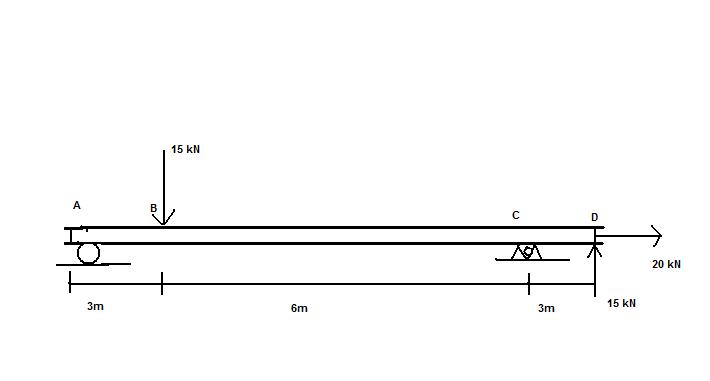# Statics: moments around beam subjected to 3 forces

• mh1985

## Homework StatementThe beam is subjected to two oppositve vertical forces and one horizontal force as shown. Determine the reactions at the supports A & C

## Homework Equations

$\sum$forces in plane = 0
moment = force x perpendicular distance

## The Attempt at a Solution

$\sum$Y = 15 + RyC + RyA - 15 = 0
$\sum$x = 20 + RxC = 0
$\sum$Moments around A = (-15 * 3) + (15 *12)+(RyC * 9)

Reaction at C in x = 20 kN
Not sure about reactions in Y at either, tried to calculate this from the moments but neither of these types of joint provide any reaction force to moments?

Last edited:

Point A is a roller. Is point C pinned?

BTW, the moment arm from A to C is 12 m according to the figure, not 15 m as shown in the calculation.

yep point C is pinned, and thanks for spotting that mistake! I still can't see how to calculate the moment reaction though because A is a roller so has no reaction to the moments?

Following on from that I get RyC = 135/9 = 15 kN... but that would mean the RyA would have to be -15kN?

Last edited:
The roller at A and the pin at C can both exert force on the beam, even though not being able to resist a moment.

Initially, static reactions at A and C are assumed to exist. Then, by applying the equations of static equilibrium, the magnitudes and directions of the reactions can be determined. Even though neither point A or point C can resist a moment, nevertheless, the sum of the moments about either point due to the applied loads and reactions must sum to zero, just like the forces do.

Moments around A = (-15 * 3) + (15 *12)+(RyC * 9) = 0

= -45 + 135 + 9 Ry at C = 0
= 90 + 9 Ry at C = 0

90/9 = Ry C = 10

But that must mean that Ry at A is negative in order for the forces in the y direction to balance?

Last edited:
You have an arithmetic mistake in your moment calculations. What is 15 * 12?

You have an arithmetic mistake in your moment calculations. What is 15 * 12?

oops that's what I get for working on it so late...180

Regardless of that error, this still doesn't make sense to me though, there are 2 vertical reactions...and they cannot be equal to 0 because the moments would not be in equilibirum.

However since the two known vertical forces are equal and opposite, both of the vertical reactions cannot be positive (upwards) or else the beam would not be in equilibrium? But how could the support provide a downwards force?

Last edited:
You must correct the arithmetic in your moment equation and calculate the reaction at C.
The roller at A can only offer an upward reaction, but the pinned connection at C can have a reaction oriented in any direction.